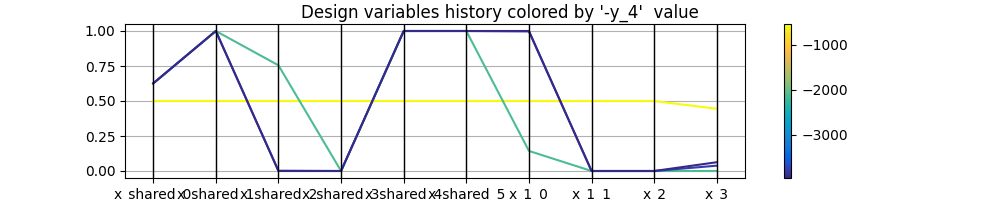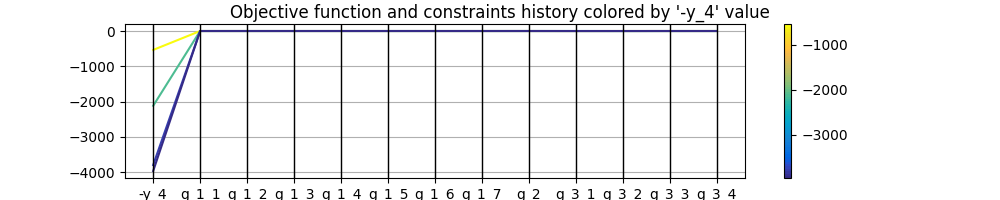# Parallel coordinates¶

In this example, we illustrate the use of the ParallelCoordinates plot on the Sobieski’s SSBJ problem.

from __future__ import absolute_import, division, print_function, unicode_literals

from future import standard_library


## Import¶

The first step is to import some functions from the API and a method to get the design space.

from gemseo.api import configure_logger, create_discipline, create_scenario
from gemseo.problems.sobieski.core import SobieskiProblem

configure_logger()

standard_library.install_aliases()


## Create disciplines¶

Then, we instantiate the disciplines of the Sobieski’s SSBJ problem: Propulsion, Aerodynamics, Structure and Mission

disciplines = create_discipline(
[
"SobieskiPropulsion",
"SobieskiAerodynamics",
"SobieskiStructure",
"SobieskiMission",
]
)


## Create design space¶

We also read the design space from the SobieskiProblem.

design_space = SobieskiProblem().read_design_space()


## Create and execute scenario¶

The next step is to build a MDO scenario in order to maximize the range, encoded ‘y_4’, with respect to the design parameters, while satisfying the inequality constraints ‘g_1’, ‘g_2’ and ‘g_3’. We can use the MDF formulation, the SLSQP optimization algorithm and a maximum number of iterations equal to 100.

scenario = create_scenario(
disciplines,
formulation="MDF",
objective_name="y_4",
maximize_objective=True,
design_space=design_space,
)
scenario.set_differentiation_method("user")
for constraint in ["g_1", "g_2", "g_3"]:
scenario.execute({"algo": "SLSQP", "max_iter": 10})


Out:

{'algo': 'SLSQP', 'max_iter': 10}


## Post-process scenario¶

Lastly, we post-process the scenario by means of the ParallelCoordinates plot which parallel coordinates plots among design variables, objective function and constraints.

scenario.post_process("ParallelCoordinates", save=False, show=True)

••Out:

<gemseo.post.para_coord.ParallelCoordinates object at 0x7fc29db186a0>


Total running time of the script: ( 0 minutes 0.815 seconds)

Gallery generated by Sphinx-Gallery